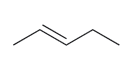Problem: Classify each of the following compounds below as  cis, trans, or not stereoisomeric:

FREE Expert Solution
86% (488 ratings)
Problem Details

Classify each of the following compounds below as  cis, trans, or not stereoisomeric:What scientific concept do you need to know in order to solve this problem?

Our tutors have indicated that to solve this problem you will need to apply the How to Recognize Cis and Trans Isomers concept. If you need more How to Recognize Cis and Trans Isomers practice, you can also practice How to Recognize Cis and Trans Isomers practice problems .

What is the difficulty of this problem?

Our tutors rated the difficulty of Classify each of the following compounds below as  cis, tran... as low difficulty.

How long does this problem take to solve?

Our expert Organic tutor, Jonathan took less than a minute to solve this problem. You can follow their steps in the video explanation above.

What professor is this problem relevant for?

Based on our data, we think this problem is relevant for Professor Gould's class at ASU.

What textbook is this problem found in?

Our data indicates that this problem or a close variation was asked in . You can also practice practice problems .